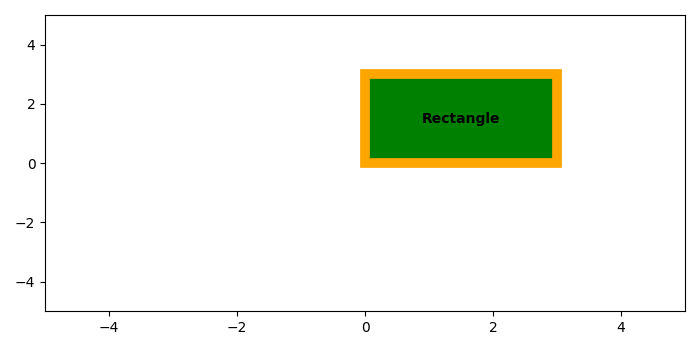# How to add a text into a Rectangle in Matplotlib?

MatplotlibPythonData Visualization

#### Python Data Science basics with Numpy, Pandas and Matplotlib

Most Popular

63 Lectures 6 hours

#### Data Visualization using MatPlotLib & Seaborn

11 Lectures 4 hours

#### MatPlotLib with Python

9 Lectures 2.5 hours

To add a text into a rectangle in matplotlib, we can add a label in annotate method at the center point of the rectangle.

## Steps

• Create a figure or activate an existing figure using figure() method.

• Add a subplot arrangement in the current axis.

• To add a rectangle in the plot, use Rectangle() class to get the rectangle object.

• Add a rectangle patch on the plot.

• To add text label in the rectangle, we can get the center value of the rectangle, i.e., cx and cy.

• Use annotate() method to place text on the rectangle.

• Limit x and y axes to get a visible rectangle.

• To display the figure, use show() method.

## Example

from matplotlib import pyplot as plt, patches
plt.rcParams["figure.figsize"] = [7.00, 3.50]
plt.rcParams["figure.autolayout"] = True
fig = plt.figure()
rectangle = patches.Rectangle((0, 0), 3, 3, edgecolor='orange',
facecolor="green", linewidth=7)
plt.show()